## A recent study suggested that 70% of all eligible voters will vote in the next presidential election. Suppose 20 eligible voters were random

Question

A recent study suggested that 70% of all eligible voters will vote in the next presidential election. Suppose 20 eligible voters were randomly selected from the population of all eligible voters. What is the probability that fewer than 11 of them will vote

in progress 0
5 months 2021-08-25T19:22:45+00:00 1 Answers 37 views 0

## Answers ( )

0.0479 = 4.79% probability that fewer than 11 of them will vote

Step-by-step explanation:

For each voter, there are only two possible outcomes. Either they will vote, or they will not. The probability of a voter voting is independent of any other voter, which means that the binomial probability distribution is used to solve this question.

Binomial probability distribution

The binomial probability is the probability of exactly x successes on n repeated trials, and X can only have two outcomes.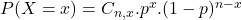In which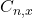is the number of different combinations of x objects from a set of n elements, given by the following formula.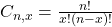And p is the probability of X happening.

70% of all eligible voters will vote in the next presidential election.

This means that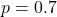20 eligible voters were randomly selected from the population of all eligible voters.

This means that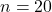What is the probability that fewer than 11 of them will vote?

This is: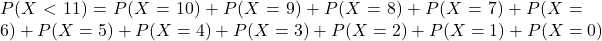In which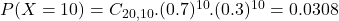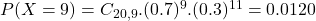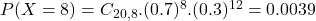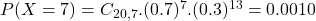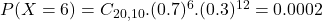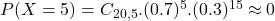The probability of 5 or less voting is very close to 0, so they will not affect the outcome. Then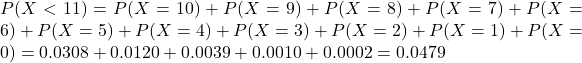0.0479 = 4.79% probability that fewer than 11 of them will vote